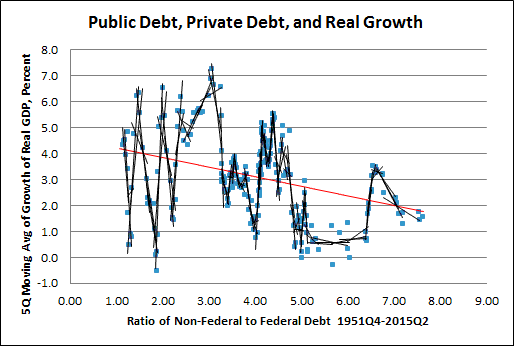## Wednesday, April 27, 2016

###Scatterplot Trends

"Learn to program" -- Bill Gates

I had some trouble determining the trends shown by scatterplots. Looked at that on 21 April ...Graph #1: Five-Year Subsets of Annual Data

... and again on 23 April.Graph #2: 12-Quarter Subsets of Quarterly Data
For the second of those two graphs I used Excel VBA to automate creating the trendlines.

Neither of the above graphs satisfied me. Wanting to improve the result as painlessly as possible, I decided to tweak the VBA code.

I added a couple variables, one to hold the start row, and one to hold the end row of the data. Since my habit is to put dates in Column A and data in Columns B and C, the start-row and end-row values are enough so that my code knows where to find the data on the spreadsheet.

Then I added a couple more variables, one for the number of data points to include in each trendline, and one for how much of an "overlap" I want from one set of data points to the next. I wanted these as variables because I wouldn't know what the graph looked like until it was done. And I wanted it to be easy to make the trendlines longer or shorter, and overlap them more or less, based on how the graphs looked.

I put all four variables together, near the top of the code. I have to go in and change the values, then run the code to change how the graph looks. But if I was doing it by hand I'd have to go into the Select Data Source form to subset the data, and then go into the Format Trendline form to finesse the trendline. I just put all the finessing in my code instead.

On both graphs above, some of the trendlines are long and some are short. It depends on the values, the RGDP growth values and the debt ratio values. On both graphs above, the data is in chronological order even though the dates are not shown on the graph. I thought I might get trendlines more equal in length if I sorted the data by the debt ratio values.Graph #3: 8-Quarter Trend Lines with 6-Quarter Overlap, Sorted on X Values
Eh, lots of the lines are still short and lots of them are still quite long. Sorting didn't help.

Still, sorting the data for a scatterplot is an interesting idea. I wouldn't want to do it for a Phillips curve, say, where there is a trade-off between x-axis values and y-axis values. But on these debt-and-growth graphs, where I'm looking at the debt ratio as cause and RGDP growth as effect, I think sorting might make sense.

If I was looking at RGDP growth over time, for example, the x values -- dates -- would be in chronological order. Why shouldn't the x values be in order even when those values are not dates? Especially if you have "cause" on the x-axis and "effect" on the y-axis.

But sorting didn't make my trendlines all the same length. Oh, well. It's the leftmost lines that strike me as long -- from the early years, when the business cycle pushed RGDP values up and down without any great "moderation". (Of course, you wouldn't know it's the early years, as there are no time values on Graph #3.) I thought I might condense the data variation by using a five-quarter moving average of the RGDP values:Graph #4: 8Q Subsets with 6Q Overlap, Sorted on X Values
Well, the lines still look long where the ratio is low, but between 5 and 6.5 on the x-axis the dots clustered nicely, and the trendlines too. Hm. I like it.

I increased the moving average from 5 to 9 quarters for the y-axis, and changed the ratio values to a 5-quarter moving average for the x-axis. Moving averages on both axes now. And now I'm starting to see a shape in those dots:Graph #5: 8Q subsets with 6Q Overlap, Sorted on X Values
I like it!

Summary: Not sure about sorting the values. But using moving averages on both the x- and the y-axis sure did make a pattern stand out.

// The Excel file. Don't believe the Google Drive preview. Download the file & open it in Excel and the graphs will be fine.